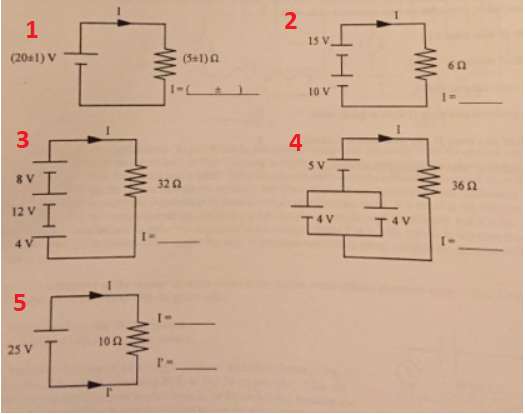# Problem: For each of the circuits shown in the accompanying figures determine the current through each resistor

###### FREE Expert Solution

In this problem, we are dealing with current, resistance, and voltage. Thus, we know that we need to use ohm's law.

Ohm's law:

87% (10 ratings)###### Problem Details

For each of the circuits shown in the accompanying figures determine the current through each resistor